Next: Analysis of the Up: Autocalibration in the presence Previous: Preliminaries

# From affine to Euclidean

The affine calibration enables to calculate the infinite homography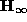between the images taken with a camera to calibrate, before and after the rigid motion. Once this infinite homography is obtained, it is possible to recover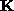thanks to the relation : eq:ginf = -1

where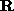is the rotation of the motion (the Euclidean frame is chosen to be the camera frame). A classical way to solve this equation was first proposed by R.Hartley [Har94] and consists to solve the equation :

eq:hartley =

where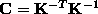is the image of the absolute conic.is then obtained by Cholesky decomposition of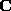. It is well known that the solutionsof eq:hartley define a 1-parameter family. This ambiguity can be eliminated if at least 2 motions with non parallel rotation axes are considered.

We propose another way to solve eq:ginf with an analysis which allows us to identify critical motions and to partially solve for calibration in these particular cases. This analysis is based on the real Jordan decomposition which has already been studied in [CDRH98] in the case of projective displacements. The approach takes into account the fact that eq:ginf definesas the conjugate of a rotation. In an appropriate frame,can be reduced to the simple form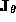: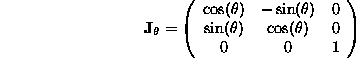Then, there exists amatrix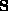such that : eq:decomp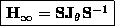This is a real Jordan decomposition of.

Bob Fisher
Mon Dec 7 13:48:06 GMT 1998